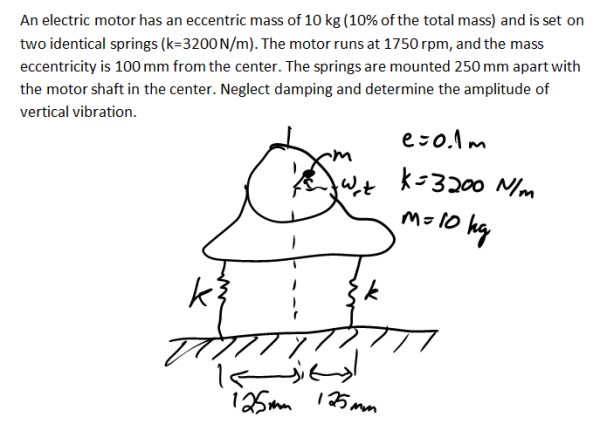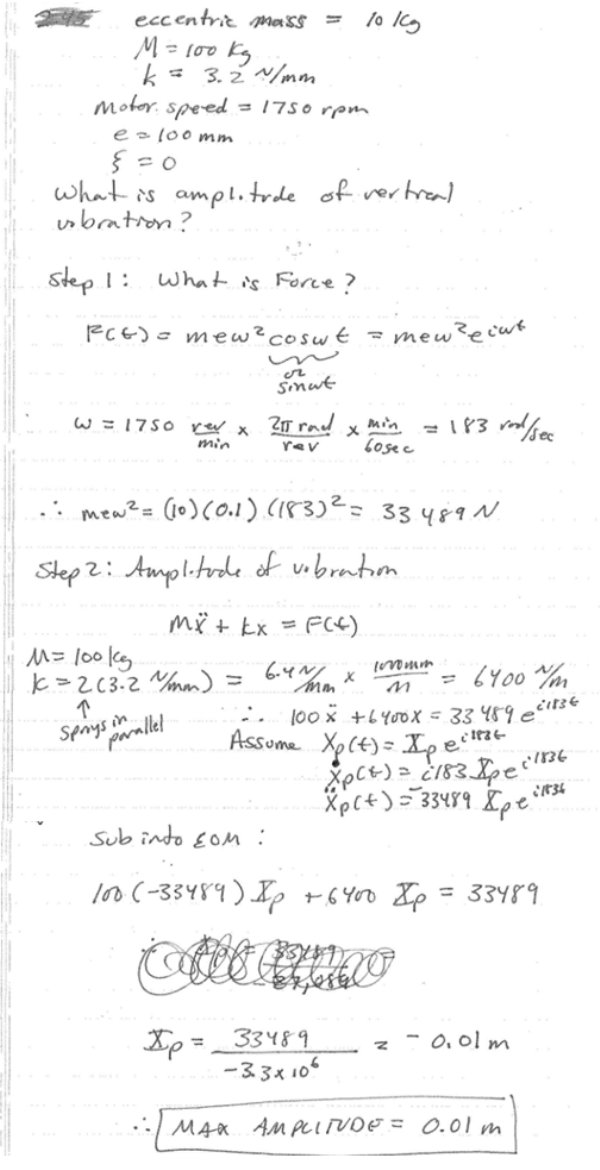An electric motor has an eccentric mass of 10 kg (10% of the total mass) and is set on two identical springs (k=3200 N/m). The motor runs at 1750 rpm, and the mass eccentricity is 100 mm from the center. The springs are mounted 250 mm apart with the motor shaft in the center. Neglect damping and determine the amplitude of vertical vibration.An electric motor has an eccentric mass of 10 kg (10% of the total mass) and is set on two identical springs (k=3200 N/m). The motor runs at 1750 rpm, and the mass eccentricity is 100 mm from the center. The springs are mounted 250 mm apart with the motor shaft in the center. Neglect damping and determine the amplitude of vertical vibration.

Vibrations Page 1 vibrations vibrations vibrations vibrations vibrations vibrations vibrations Vibrations Page 2